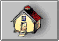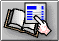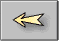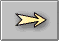## Introduction to Controlled Impedance PCBs

The increase in processor clock speed and component switching speed on modern PCBs means that the interconnecting paths between components (i.e. PCB traces) can no longer be regarded as simple conductors.

At fast switching speeds or high frequencies (i.e. for digital edge speeds faster than 1ns or analog frequencies greater than 300MHz) PCB traces must be treated as transmission lines; i.e. for stable and predictable high speed operation the electrical characteristics of PCB traces and the dielectric of the PCB must be controlled.

One critical parameter is the characteristic impedance of the PCB trace (the ratio of voltage to current of a wave moving down the signal transmission line); this will be a function of the physical dimensions of the trace (e.g. trace width and thickness) and the dielectric constant of the PCB substrate material and dielectric thickness. The impedance of a PCB trace will be determined by its inductive and capacitive reactance, resistance and conductance. PCB impedances will typically range from 25Ω to 120Ω.

In practice a PCB transmission line typically consists of a line conductor trace, one or more reference planes and a dielectric material. The transmission line, i.e. the trace and planes, form the controlled impedance.

The PCB will frequently be multi-layer in fabrication and the controlled impedance can be constructed in several ways. However, whichever method is used the value of the impedance will be determined by its physical construction and electrical characteristics of the dielectric material:

The width and thickness of the signal trace

The height of the core or pre-preg material either side of the trace

The configuration of trace and planes

The dielectric constant of the core and pre-preg material